Next: Solid sphere with convection. Up: EXAMPLES, TEMPERATURE FROM GF Previous: 1D Slab heated at

## Solid cylinder with internal heating.

A long cylinder is initially at zero temperature and the boundary at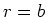is maintained at zero temperature. Find the temperature in the cylinder resulting from spatially uniform internal energy generation,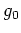(W/m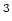).

The temperature satisfies the following equations: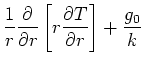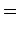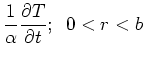(18)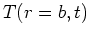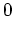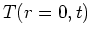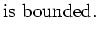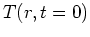This case is number R01B0T0G1. The GF solution equation for the temperature contains only the internal heating term: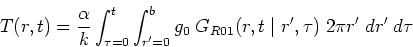(19)

The large-time form of the GF for this case is given by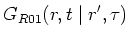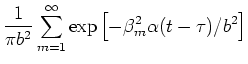(20)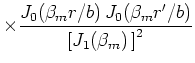(21)

where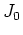and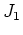are Bessel functions and the eigenvalues given by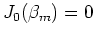. The two integrals in the above solution will be considered one at a time. The spatial integral over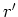acts on oneterm, and it may be simplified by the substitution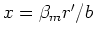and then evaluated as shown below: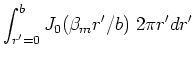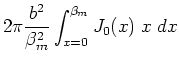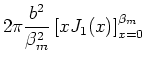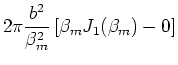The time integral acts only on the exponential term of the series for the GF, given by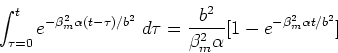(22)

Then the above two integrals can be combined with the entire GF solution to give: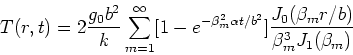(23)

For numerical evaluation, the steady term should be computed separately and substituted for the slowly converging series term (the term without the exponential). When the steady-state portion of the solution is substituted (see below), the temperature is given by: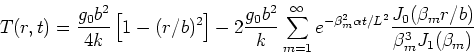(24)

This form of the solution converges rapidly for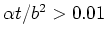.

Steady Solution. Next the steady solution for the solid sphere with internal heating will be derived. The steady temperature satisfies the following differential equation: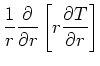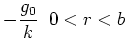(25)The general solution may be found by integrating the differential equation twice: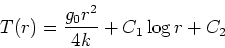The constants of integration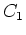and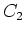may be determined by applying the boundary condtions. For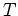to be bounded at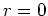requires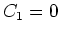. Atthe boundary condition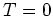then determines. Then the steady temperature is given by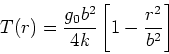Next: Solid sphere with convection. Up: EXAMPLES, TEMPERATURE FROM GF Previous: 1D Slab heated at
2004-01-31